Theories of light

In the seventeenth century two rival theories of the nature of light were proposed, the wave theory and the corpuscular theory.

The Dutch astronomer Huygens (1629-1695) proposed a wave theory of light. He believed that light was a longitudinal wave, and that this wave was propagated through a material called the 'aether'. Since light can pass through a vacuum and travels very fast Huygens had to propose some rather strange properties for the aether: for example; it must fill all space and be weightless and invisible. For this reason scientists were sceptical of his theory.

In 1690 Newton proposed the corpuscular theory of light. He believed that light was shot out from a source in small particles, and this view was accepted for over a hundred years.

The quantum theory put forward by Max Planck in 1900 combined the wave theory and the particle theory, and showed that light can sometimes behave like a particle and sometimes like a wave. You can find a much fuller consideration of this in the section on the quantum theory.

Wave theory of Huygens

As we have seen, Huygens considered that light was propagated in longitudinal waves through a material called the aether. We will now look at his ideas more closely.

Huygens published his theory in 1690, having compared the behaviour of light not with that of water waves but with that of sound. Sound cannot travel through a vacuum but light does, and so Huygens proposed that the aether must fill all space, be transparent and of zero inertia. Clearly a very strange material!

Even at the beginning of the twentieth century, however, scientists were convinced of the existence of the aether. One book states 'whatever we consider the aether to be there can be no doubt of its existence'.

We now consider how Huygens thought the waves moved from place to place.
Consider a wavefront initially at position W, and assume that every point on that wavefront acts as a source of secondary wavelets. (Figure 1 shows some of these secondary sources). The new wavefront W1 is formed by the envelope of these secondary wavelets since they will all have moved forward the same distance in a time t (Figure 1).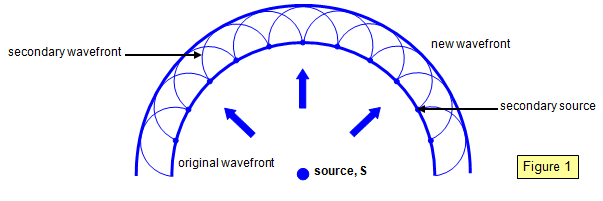There are however at least two problems with this idea and these led Newton and others to reject it:
(a) the secondary waves are propagated in the forward direction only, and
(b) they are assumed to destroy each other except where they form the new wavefront.

Newton wrote: 'If light consists of undulations in an elastic medium it should diverge in every direction from each new centre of disturbance, and so, like sound, bend round all obstacles and obliterate all shadow.' Newton did not know that in fact light does do this, but the effects are exceedingly small due to the very short wavelength of light.

Huygens' theory also failed to explain the rectilinear propagation of light.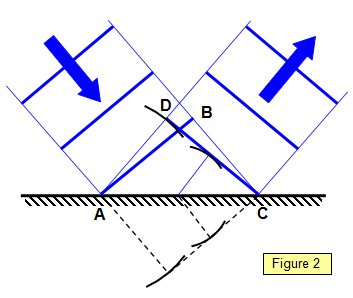The reflection of a plane wavefront by a plane mirror is shown in Figure 2. Notice the initial position of the wavefront (AB), the secondary wavelets and the final position of the wavefront (CD). Notice that he shape of the wavefront is not affected by reflection at a plane surface.
The lines below the mirror show the position that the wavefront would have reached if the mirror had not been there.

We will now show how Huygens' wave theory can be used to explain reflection and refraction and the laws governing them.

(a) Reflection

Consider a parallel beam of monochromatic light incident on a plane surface, as shown in Figure 3. The wave fronts will be plane both before and after reflection, since a plane surface does not alter the shape of waves falling on it.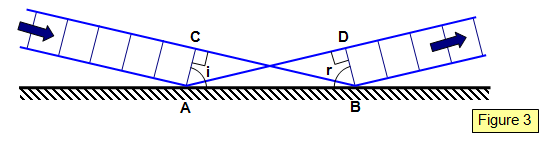Consider a point where the wavefront AC has just touched the mirror at edge A. While the light travels from A to D, that from C travels to B. The new envelope for the wavefront AC will be BD after reflection.

Therefore: AD = CB, Angle ACB = angle ADB = 90o, AB is common

Therefore ΔACB and ΔBDA are similar and so angle CAB = angle BAD. Therefore i = r and the law of reflection is proved.

(b) Refraction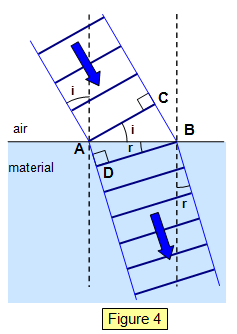Consider a plane monochromatic wave hitting the surface of a transparent material of refractive index n. The velocity of light in the material is cm and that in air ca. Now in Figure 4,

CB = AB sin i

The same argument applies about the new envelope as in the case of reflection:

time to travel CB = CB/ca = AB sin i/ca

But these are equal and therefore:

ca/cm = sin i/sin r = anm.

This is Snell's law, and it was verified later by Foucault and others.

Notice that since the refractive index of a transparent material is greater than 1, Huygens' theory requires that the velocity of light in air should be greater than that in the material.

Corpuscular theory of Newton

Newton proposed that light is shot out from a source as a stream of particles. He argued that light could not be a wave because although we can hear sound from behind an obstacle we cannot see light - that is, light shows no diffraction. He stated that particles of different colours should be of different sizes, the red particles being larger than the blue.

Since these particles are shot out all the time, according to Newton's theory, the mass of the source of light must get less!

We can use Newton's theory to deduce the laws of reflection and refraction.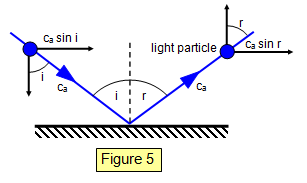(a) Reflection

Consider a particle of light in collision with a mirror. The collision is supposed to be perfectly elastic, and so tile component of velocity perpendicular to the mirror is reversed while that parallel to the mirror remains unaltered.

Component of velocity before collision parallel to the mirror = ca sin i
Component of velocity after collision parallel to the mirror = ca sin r
Therefore:
ca sin i = ca sin r

and so the law of reflection is proved.

(b) Refraction

Newton assumed that there is an attraction between the molecules of a solid and the particles of light, and that this attraction acts only perpendicularly to the surface and only at very short distances from the surface. (He explained total internal reflection by saying that the perpendicular component of velocity was too small to overcome the molecular attraction.) This has the effect of increasing the velocity of the light in the material.
Let the velocity of light in air be ca and the velocity of light in the material in Figure 6 be cm.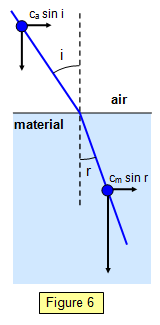The velocity parallel to the material is unaltered and therefore:
casin i = cmsin r
Therefore:
cm/ca = sini/ sinr = anm

This ratio is the refractive index, but because n > 1 the velocity of light in the material must be greater than that in air. Newton accepted this result and other scientists preferred it to that of Huygens, mainly because of Newton's eminence.

A problem of the corpuscular theory was that temperature has no effect on the velocity of light, although on the basis of this theory we would expect the particles to be shot out at greater velocities as the temperature rises.

Classical and modern theories of light

It is interesting to compare the two classical theories of light and see which phenomena can be explained by each theory. The following table does this.

 Wave theory Corpuscular theory Reflection Reflection Refraction Photoelectric effect Diffraction Interference

Notice that neither theory can account for polarisation, since for polarisation to occur the waves must be transverse in nature.

Modem theories
Twentieth-century ideas have led us to believe that light is:
(a) a transverse electromagnetic wave with a small wavelength, and
(b) emitted in quanta or packets of radiation of about 10-8 s duration with abrupt phase changes between successive pulses.

A VERSION IN WORD IS AVAILABLE ON THE SCHOOLPHYSICS USB